you are here:/ News & Insights / Engineering Advantage Blog / Understanding the Finite Element Iterative Solver - Part II

# Understanding the Finite Element Iterative Solver - Part IIApril 17, 2015 By: Eric Stamper

Part I of this blog post defined a sample problem and provided a brief discussion on its setup. We're now continuing on with that problem to illustrate the concepts behind the conjugate gradient (CG) method. The original problem described is shown here again: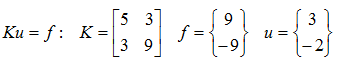The idea behind an iterative solver is to obtain the answer by taking an initial guess at the solution. This initial guess will not likely be correct (i.e. there will be some error "e"); therefore it will need to be adjusted (an iteration) to reach the minimum point (i.e. the solution). The CG method effectively points the "adjustment" in the direction of the solution or the direction of minimum error.

The fastest path in this context is with two vectors d(i) and d(j) that are "conjugate" (a type of orthogonality condition):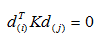The condition that e(i+1) must be conjugate to d(i) is then formed, which is equivalent to finding the minimum point along the search direction d(i).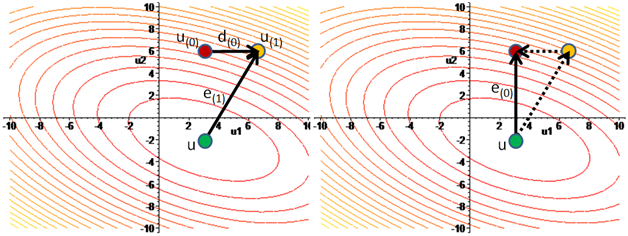The idea behind this approach is to find the search directions (d(0),...,d(n-1)) that each line up to the solution "u" in that direction. This process is shown in the above two images. Starting with the initial guess (u(0)), a step is taken in a direction (d(0)). The point u(1) is constrained by the requirement that e(1) is conjugate to d(0). The initial error e(0) is then the sum of the conjugate components shown on the right as dashed lines, of which, each component is eliminated each iteration. In our case we have two unknowns, therefore the maximum number of iterations needed to find the solution is n=2. This is accomplished by decreasing the error term each iteration until e(n)=0.

This is the conjugate method; however for practical problems with millions of degrees of freedom that need to be solved, we wouldn't be able to afford millions of iterations. As a method around this, the error that is minimized is rewritten as a linear combination of the search directions; or eigenvectors: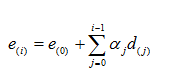The process of determining "u" component by component can also be viewed as a process of reducing the error term component by component, and after n iterations, every component is cut away, and e(n)=0.

We're then looking to form a polynomial that represents the series of eigenvectors d(j) with coefficients (eigenvalues) α(j), and the CG method chooses coefficients that minimize the error e(i). How quickly the convergence is achieved is based on how close the polynomial can be to zero for each eigenvalue.

This can be determined by the ratio of the maximum eigenvalue to the minimum eigenvalue or what's referred to as the condition number, k. The convergence of the CG method improves as the condition number of the matrix decreases. The more ill-conditioned the matrix (i.e. the larger its condition number), the slower the convergence per iteration.

The relationship between the error being minimized and rate of convergence, can be expressed in the following form: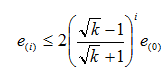This is easier to visualize by looking at a plot of just the term in the parentheses for the first few iterations as shown below. The error term diminishes as the iterations "i" grow, and the lower the condition number, the faster the error decreases per iteration (the rate of convergence).

## iterative2_1.pngFor a condition number of one (i.e. the eigenvalues are equal), the rate of convergence is zero or instant.

For our problem, the condition number based on the eigenvalues of K is: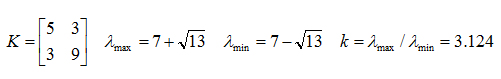Therefore; the error term after the first iteration is no greater than 0.55 times its initial value. The second iteration it's no greater than 0.15 and so on until the error is acceptably small.

When the eigenvalues are grouped together the CG method converges more quickly (e.g. it's easier to form a polynomial that fits closely spaced points). Therefore; if the range is smaller or the eigenvalues are better clustered together, the problem can be solved faster, which relates to a problem with a lower condition number.

Preconditioning is a technique for improving the condition number of a matrix, thereby improving the rate of convergence. Preconditioning transforms the original problem into a new form with a better condition number and indirectly solves the original problem. This transformation process can take the form of the following: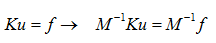If the eigenvalues of M-1K result in a better condition number than K, the transformed problem can be solved faster than the original problem.

The best preconditioner "M" would be M=K, since the condition number would be one; however you'd just be solving for Mu=f, at which point you could just use a direct solver. The preconditioner therefore should approximate K well enough to improve the rate of convergence, which leaves many possible options. The simplest being a diagonal matrix equal to the diagonal terms of K, (used by the JCG solver in ANSYS). Another option is to factor K into a lower triangular matrix "L" in the form of LLT (the ICCG solver). The PCG solver in ANSYS employs a sophisticated preconditioner as well, but regardless of the choice, the CG method greatly benefits from a preconditioner which is why it's used in finite element codes.

Hopefully this helps clear up some of the “mystery” behind the iterative solvers used for very large FEA problems. I would like to learn more about your experiences with this approach, so please add a comment.